# Texas Go Math Grade 6 Lesson 1.2 Answer Key Comparing and Ordering integers

Refer to our Texas Go Math Grade 6 Answer Key Pdf to score good marks in the exams. Test yourself by practicing the problems from Texas Go Math Grade 6 Lesson 1.2 Answer Key Comparing and Ordering integers.

## Texas Go Math Grade 6 Lesson 1.2 Answer Key Comparing and Ordering integers

Reflect

Question 1.
Analyze Relationships Explain what the data tell you about the win/loss records of the teams in the league.
Given data tells us:

• Whether each team has more wins or more losses.
• It tells us which team has most wins and which least.

Graph the values in each table on a number line. Then list the numbers in order from greatest to least.

Question 2.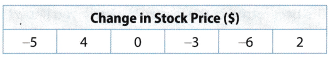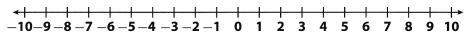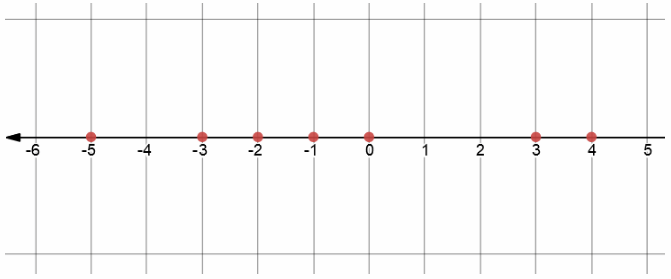Number 4 is greater than 3 which is greater than 0 which is greater than – 1
which is greater than – 2 which is greater than – 3 which is greater than
Therefore, we write:
4 > 3 > 0 > – 1 > – 2 > – 3 > – 5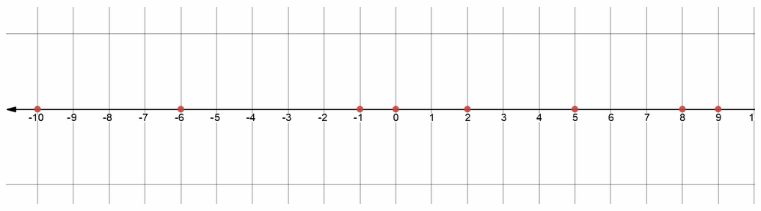Number 9 is greater than 8 which is greater than 5 which is greater than 2
which is greater than 0 which is greater than – 1 which is greater than – 6
which is greater than – 10
Therefore, we write:
9 > 8 > 5 > 2 > 0 > – 1 > – 6 > – 10

Compare. Write > or <. Use the number line to help you.

Question 4.
– 10 ________ – 2
Number – 10 is smaller than – 2
Therefore, we write:
– 10 < – 2

Question 5.
– 6 ________ 6
Number – 6 is smaller than 6
Therefore, we write:
– 6 < 6

Question 6.
– 7 ________ – 8
Number – 7 is greater than – 8
Therefore, we write:
– 7 > – 8

Question 7.
Write two inequalities to compare – 2 and – 18.
Number – 18 is smaller than – 2
Therefore, we write:
– 18 < – 2

Write two inequalities to compare 39 and – 39.
Number – 39 is smaller than 396
Therefore, we write:
– 39 < 39

Question 1.
a. Graph the temperature for each city on the number line. (Explore Activity)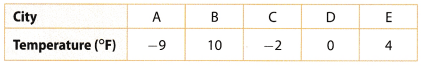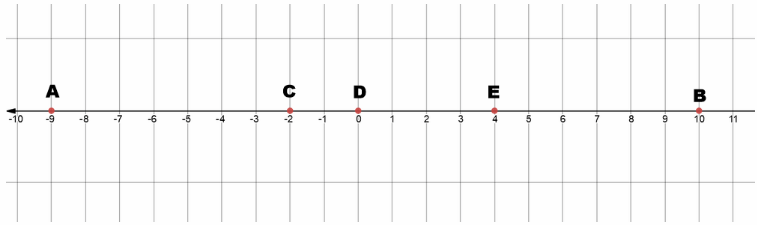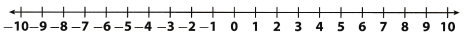b. Which city was coldest? ______________
Coldest city was A

c. Which city was warmest? _____________
Warmest city was B

List the numbers in order from least to greatest. (Example 1)

Question 2.
4, – 6, 0, 8, – 9, 1, – 3
Number – 9 is smaller than – 6 which is smaller than – 3 which is smaller than 0
which is smaller than 1 which is smaller than 4 which is smaller than 8
Therefore, we write
– 9 < – 6 < – 3 < 0 < 1 < 4 < 8

Question 3.
– 65, 34, 7, – 13, 55, 62, – 7
Number – 65 is smaller than – 13 which is smaller than – 7
which is smaller than 7 which is smaller than 34 which is smaller than 55 which is smaller than 62
Therefore, we write:
– 65 < – 13 < – 7 < 7 < 34 < 55 < 62

Go Math Grade 6 Lesson 1.2 Comparing and Ordering Integers Question 4.
Write two inequalities to compare – 17 and – 22.
Number – 17 is greater than – 22
Therefore, we write:
– 17 > – 22

Number – 22 is smaller than – 17
Therefore, we write:
– 22 < – 17

Compare. Write < or >.

Question 5.
– 9 _______ 2
Number – 9 is smaller than 2
Therefore, we write:
– 9 < 2

Question 6.
0 _______ 6
Number 0 is smaller than 6
Therefore, we write:
0 < 6

Question 7.
3 _______ – 7
Number 3 is greater than – 7
Therefore, we write:
3 > – 7

Question 8.
5 _______ – 10
Number 5 is greater than – 10
Therefore, we write:
– 5 > – 10

Question 9.
– 1 _______ – 3
Number – 1 is greater than – 3
Therefore, we write:
– 1 > – 3

Question 10.
– 8 _______ – 4
Number – 8 is smaller than – 4
Therefore, we write:
– 8 < – 4

Lesson 1.2 Identifying Integers and Their Opposites Answer Key Question 11.
– 4 _______ 1
Number – 4 is smaller than 1
Therefore, we write:
– 4 < 1

Question 12.
– 2 _______ – 6
Number – 2 is greater than – 6
Therefore, we write:
– 2 > – 6

Question 13.
Compare the temperatures for the following cities. Write < or >.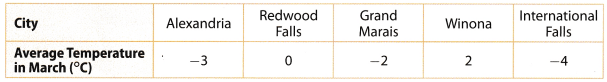a. Alexandria and Winona
Temperature in Alexandria is – 3° and temperature in Winona is 2° so
Number – 3 is smaller than 2
Therefore we write:
– 3 < 2

b. Redwood Falls and International Falls
Temperature in Redwood Falls is 0° and
temperature in International Falls is – 4° s0
Number 0 is greater than – 4
Therefore, we write:
0 > – 4

Essential Question Check-In

Question 14.
How can you use a number line to compare and order numbers?
We can use number line to determine which number is greater or smaller!
The farthest number on left is least and farthest number on right is greatest!
Analogically, we can order all the other given numbers!

Question 15.
Multiple Representations A hockey league tracks the plus-minus records for each player. A plus-minus record is the difference in even strength goals for and against the team when a player is on the ice. The following table lists the plus-minus values for several hockey players.a. Graph the values on the number line.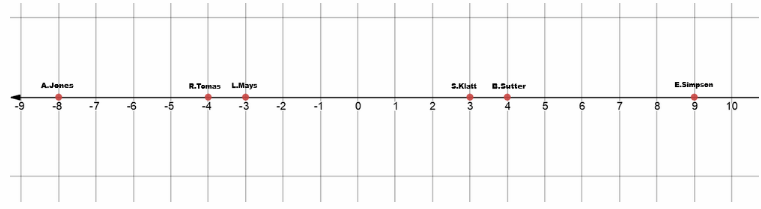b. Which player has the best plus-minus record? __________
Viewing the number line we can determine which player has the best plus-minus record!
It is: E.Simpson

Astronomy The table lists the average surface temperature of some planets. Write an inequality to compare the temperatures of each pair of planets.Uranus and Jupiter _____________________
The temperature on Uranus is – 197°C and the temperature on Jupiter is – 110°C 50
Temperature – 197°C is smaller than – 110°C
Therefore, we write:
– 197°C < – 110°C

Question 17.
Mercury and Mars _____________________
Temperature on Mercury is 167°C and temperature on Mars is – 65°C so
Temperature 167°C is greater than – 65°C
Therefore, we write:
167°C > – 65°C

Question 18.
Arrange the planets in order of average surface temperature from greatest to least. _____________________
We write temperatures from table and separate theta in an order from greatest to smallest average surface temperature.

Temperature 167° is greater than 15° which is greater than – 65° which is greater than – 110°
which is greater than – 197° which is greater than 200°

Therefore, we write:
167° > 15° > 65° > – 110° > 197° > 200°

Question 19.
Represent Real-World Problems For a stock market project, five students each invested pretend money in one stock. They tracked gains and losses in the value of that stock for one week. In the following table, a gain is represented by a positive number and a loss is represented by a negative number.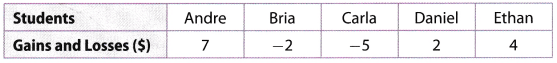Graph the students results on the number line. Then list them in order from least to greatest.

a. Graph the values on the number line.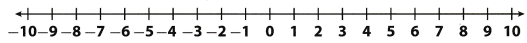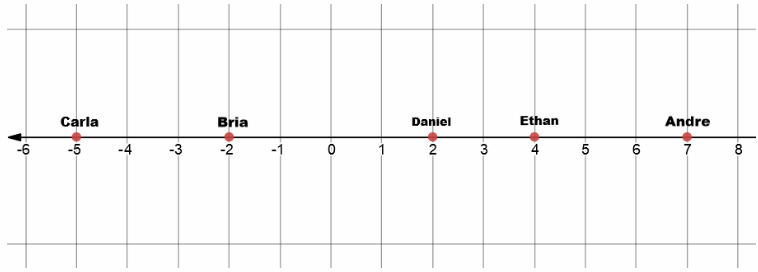b. The results listed from least to greatest are ______________ .
We can simply take a look to a graph above!
Just read student names connected with values from left to right
Doing that, we get:
– 5< – 2 < 2 < 4 < 7

Geography The table lists the lowest elevation for several countries. A negative number means the elevation is below sea level, and a positive number means the elevation is above sea level. Compare the lowest elevation for each pair of countries. Write < or >.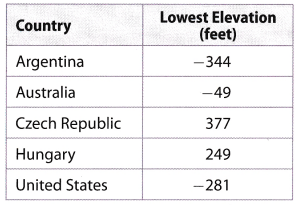Go Math Grade 6 Lesson 1.2 Practice and Homework Question 20.
Argentina and the United States ______________
Argentina has the lowest elevation of – 344 feet and United States has a Lowest elevation of – 281 feet.
Therefore, we can say:
Argentina’s lowest elevation is lower than Unites States lowest elevation as number – 344 is smaller than – 281 .
And we write:
– 344 < – 281
Argentina’s lowest elevation is lower than United State’s

Question 21.
Czech Republic and Hungary ______________
Czech Republic has lowest elevation of 377 fret and Hungary has lowest elevation of 249 feet.
Therefore, we can say:
Czech Republic’s lowest elevation is higher than Hungary’s lowest elevation as number 377 is greater than 249 .
And We write:
377 > 249
Czech Republic’s Lowest elevation is higher than Hungary’s

Question 22.
Hungary and Argentina ______________
Hungary has lowest elevation of 249 feet and Argentina has lowest eLevation of – 344 feet.

Therefore, we can say:
Hungary’s lowest elevation is higher than Argentina’s lowest elevation as number 249 is greater than – 344
And we write:
249 > – 344
Hungary’s lowest elevation is higher than Argentina’s!

Question 23.
Which country in the table has the lowest elevation? _______________
When we are asked to find the lowest elevation of all countries, we just compare numbers connected to each country and find the smallest one and that will be our solution!

Therefore, we can say:
Number – 344 is smallest number of given ones!
So, country that has lowest elevation is Argentina.
Lowest elevation is Argentina!

Question 24.
Analyze Relationships There are three numbers a, b, and c, where a > b and b > c. Describe the positions of the numbers on a number line.
If we have some random 3 numbers on a number line a, b and c.
We know that a > b and b > c
We can conclude that a > c
So, we will have them placed:
a – farthest right
b – in middle
c – farthest left

H.O.T. Focus on Higher Order Thinking

Texas Go Math Lesson 1.2 Prime Factorization Answers 6th Grade Question 25.
Critique Reasoning At 9 A.M. the outside temperature was – 3°F. By noon, the temperature was – 12°F. Jorge said that it was getting warmer outside. Is he correct? Explain.
Jorge is, surely wrong because the temperature actually decreased by noon
We can write these temperatures as numbers and compare them to prove our theory:
Number – 3 is greater than – 12
Therefore, we write:
– 3 > – 12
So temperature actually decreased from – 3°F to – 12°F

Question 26.
Problem-Solving Golf scores represent the number of strokes above or below par. A negative score means that you hit a number below par while a positive score means that you hit a number above par. The winner in golf has the lowest score. During a round of golf, Angela’s score was – 5 and Lisa’s score was – 8. Who won the game? Explain.
We can watch the given number of strokes as ordinary numbers and conclude:
Number – 5 is greater than – 8
Therefore, we write:
– 5 > – 8
So the winner is Lisa since we are Looking for a smaller value!
Lisa won the game!

Go Math Lesson 1.2 6th Grade Question 27.
Look for a Pattern Order – 3, 5, 16, and – 10 from least to greatest. Then order the same numbers from closest to zero to farthest from zero. Describe how your lists are similar. Would this be true if the numbers were – 3, 5, – 16 and – 10?